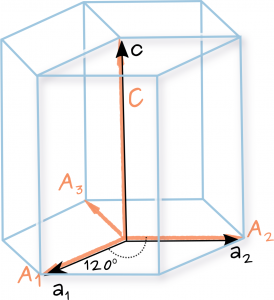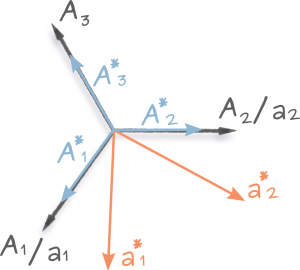This page was built to translate between Miller and Miller-Bravais indices, to calculate the angle between given directions and the plane on which a lattice vector is normal to for both cubic and hexagonal crystal structures. For more information on crystallographic computations in the real and reciprocal space check this tutorial (pdf). We also recommend the following excellent textbook: “Structure of Materials: An Introduction to Crystallography, Diffraction and Symmetry” by M. De Graef and M. E. McHenry.

Background...
The hexagonal system is more conveniently described by 4 basis vectors (Miller-Bravais index notation), 3 of which are co-planar and therefore, not linearly independent. Hence, the tendency to describe planes in hexagonal crystal using the Miller-Bravais system $$(hkil)$$ where $$i=-(h+k)$$ and can be omitted in writting $$(hk.l)$$. Note that $$i$$ is determined from $$h$$ and $$k$$ and therefore not a user modifiable variable.

The 1st block, (hkl)⇔(hkil) converts Miller indices describing a set of planes to the equivalent Miller-Bravais indices through the following relationship:

$$i=-(h+k) \quad h=h \quad k=k \quad l=l.$$

## 1) Hexagonal Miller plane indices to Miller-Bravais indices and back: (hkl)⇔(hkil)

( )

( )

Background...
Unlike the notation for planes which remains mostly unaffected but the index $$i$$ when translating between Miller and Miller-Bravais, the notation for direction requires a little more care.

For instance the crystallographic direction described in Miller indices as $$[uvw]$$ is given by the translation vector $$\mathbf{t}= u\mathbf{a_1} + v\mathbf{a_2} + w\mathbf{c}$$ in terms of the three basis vectors of the hexagonal lattice $$\{\mathbf{a_1}, \mathbf{a_2}, \mathbf{c}\}$$. In terms of four vectors the same translation vector will be  $$\mathbf{t} = U\mathbf{A_1} + V\mathbf{A_2} + T\mathbf{A_3} + W\mathbf{C}$$ and will be described by the Miller-Bravais indices as $$[UVTW]$$ .  The three vectors basis define the  primitive hexagonal (hP) rhomboid prism cell (see drawing). The four vectors define a hexagonal prism cell made out of three hP cell. The following relations are true about the two basis sets: $$\mathbf{A_1}=\mathbf{a_1}, \quad \mathbf{A_2}=\mathbf{a_2}, \quad \mathbf{A_3}=-(\mathbf{a_1} + \mathbf{a_2}) , \quad \mathbf{C}=\mathbf{c}$$.

The relations between the translation vector components in the two notation systems follows from the equality:

$$U\mathbf{A_1} + V\mathbf{A_2} + T\mathbf{A_3} + W\mathbf{C} = u\mathbf{a_1} + v\mathbf{a_2} + w\mathbf{c}$$

The 2nd block [uvw]⇔[UVTW] converts direction vectors written in Miller indices to Miller-Bravais indices and back through the following formulas:Hexagonal primitive cell made out of three hP unit cell together with the basis vectors describing them.

Miller $$[uvw]$$ ⇒ Miller-Bravais $$[UVTW]:$$

$$U=\frac{1}{3}(2u-v) \hspace{0.5cm}$$

$$V=\frac{1}{3}(2v-u)\hspace{0.5cm}$$

$$T=-(U+V) \hspace{0.5cm}$$

$$W=w \hspace{0.5cm}$$

Miller-Bravais $$[UVTW]$$ ⇒ Miller $$[uvw]:$$

$$u = 2U+V \hspace{0.5cm}$$

$$v = 2V+U \hspace{0.5cm}$$

$$w=W\hspace{0.5cm}$$

As before, $$T$$ is not independent and cannot be modified by the user.

## 2) Hexagonal Miller direction indices to Miller-Bravais indices and back: [uvw]⇔[UVTW]

[ ]

[ ]

Background...

The dot product between two vectors $$\mathbf{p} = p_i \mathbf{a}_i$$ and $$\mathbf{q} = q_i \mathbf{a}_i$$ defined in a crystal system with lattice parameters $$\{a, b, c, \alpha, \beta, \gamma\}$$ and basis vectors $$\{\mathbf{a_1}, \mathbf{a_2}, \mathbf{a_3}\}$$ is given generally by:

$$\mathbf{p} \cdot \mathbf{q} = |\mathbf{p}| |\mathbf{q}| \cos{\theta} = p_i \mathbf{a}_i \cdot q_j \mathbf{a}_j = p_i g_{ij} q_j$$

where $$g_{ij}$$ is the direct matrix tensor defined as:

$$\begin{equation} g = \begin{bmatrix} \mathbf{a_1} \cdot \mathbf{a_1} & \mathbf{a_1} \cdot \mathbf{a_2} & \mathbf{a_1} \cdot \mathbf{a_3} \\ \mathbf{a_2} \cdot \mathbf{a_1} & \mathbf{a_2} \cdot \mathbf{a_2} & \mathbf{a_2} \cdot \mathbf{a_3} \\ \mathbf{a_3} \cdot \mathbf{a_1} & \mathbf{a_3} \cdot \mathbf{a_2} & \mathbf{a_3} \cdot \mathbf{a_3} \end{bmatrix} = \begin{bmatrix} a^2 & a b \cos{\gamma} & a c \cos{\beta} \\ b a \cos{\gamma} & b^2 & b c \cos{\alpha} \\ c a \cos{\beta} & c b \cos{\alpha} & c^2 \end{bmatrix} \end{equation} \quad $$

The angle $$\theta$$ between the vectors $$\mathbf{p}$$ and $$\mathbf{q}$$ is then:

$$\theta = \cos^{-1}{\frac{p_i g_{ij} q_j}{\sqrt{p_i g_{ij} p_j} \sqrt{q_i g_{ij} q_j}}} \quad $$

For a cubic crystal with lattice parameters $$\{a, a, a, 90, 90, 90\}$$ the direct metric tensor reduces to:

$$g^{cub} = \begin{bmatrix} a^2 & 0 & 0 \\ 0 & a^2 & 0 \\ 0 & 0 & a^2 \end{bmatrix} = a^2 \begin{bmatrix} 1 & 0 & 0 \\ 0 & 1 & 0 \\ 0 & 0 & 1 \end{bmatrix} = a^2 \delta{ij}$$

Which also means that the angle formula reduces to:

$$\theta = \cos^{-1}{\frac{p_i q_i}{\sqrt{p_i p_i} \sqrt{q_i q_i}}}$$

which is the formula we know from the Cartesian system.
The 3rd block, [u1 v1 w1] ∠ [u2 v2 w2], uses the dot product formula to calculate the cosine between two vectors $$\mathbf{V}_1=u_1\mathbf{a_1} +v_1\mathbf{a_2} + w_1\mathbf{a_3}$$ and $$\mathbf{V_2}=u_2\mathbf{a_1} +v_2\mathbf{a_2} + w_2\mathbf{a_3}$$ defined in a cubic crystal. The resulting angle is shown in both degrees and rads:

$$cos(\theta)=\displaystyle\frac{u_{1}u_{2}+v_{1}v_{2}+w_{1}w_{2}}{\sqrt{(u_1^2+v_1^2+w_1^2)(u_2^2+v_2^2+w_2^2)}}$$

## 3) Angle between two cubic directions: [u1 v1 w1] ∠ [u2 v2 w2]

[ ] [ ]

=    =    =

Background...
For a hexagonal crystal with lattice parameters $$\{a, a, c, 120, 120, 90\}$$ the direct metric can be written from eq. :

$$g^{hex} = \begin{bmatrix} a^2 & -a^2/2 & 0 \\ -a^2/2 & a^2 & 0 \\ 0 & 0 & c^2 \end{bmatrix}$$

The magnitude of a vector, $$\mathbf{p}$$, defined in a hexagonal crystal system, $$\mathbf{p}=p_1\mathbf{a_1}+p_2\mathbf{a_2}+p_3\mathbf{c}$$ is then:

$$|\mathbf{p}| = \sqrt{p_i\,\, g^{hex}_{ij} \,\, p_j} = \sqrt{(a^2(p_1^2-p_1 p_2 +p_2^2) + c^2p_3^2)}$$

If we now consider two directions $$[u_1 v_1 w_1]$$ and $$[u_2 v_2 w_2]$$ defined this time in a hexagonal crystal with basis vectors $$\mathbf{a}_1, \mathbf{a}_2, \mathbf{c}$$, then the angle between can be calculated from eq.  to be:

$$cos(\theta)=\displaystyle\frac{a^2\left(u_{1}u_{2} + v_{1}v_{2} -\frac{1}{2}(u_1 v_2+v_1 u_2)\right)+ c^2 w_{1}w_{2}}{\sqrt{a^2(u_1^2-u_1 v_1 + v_1^2) + c^2 w_1^2 } \sqrt{a^2(u_2^2 -u_2v_2+v_2^2) + c^2 w_2^2}}$$

The 4th block, [u1 v1 w1] ∠ [u2 v2 w2], calculates the angle between two hexagonal crystal direction given in Miller indices.

A similar equation can be derived for direction given in Miller-Bravais indices. The direct metric tensor for a hexagonal crystal system defined with the four-basis vectors is:

$$^{hex}G=\begin{bmatrix} \mathbf{A_1} \cdot \mathbf{A_1} & \mathbf{A_1} \cdot \mathbf{A_2} & \mathbf{A_1} \cdot \mathbf{A_3} & \mathbf{A_1} \cdot \mathbf{C} \\ \mathbf{A_2} \cdot \mathbf{A_1} & \mathbf{A_2} \cdot \mathbf{A_2} & \mathbf{A_2} \cdot \mathbf{A_3} & \mathbf{A_2} \cdot \mathbf{C} \\ \mathbf{A_3} \cdot \mathbf{A_1} & \mathbf{A_3} \cdot \mathbf{A_2} & \mathbf{A_3} \cdot \mathbf{A_3} & \mathbf{A_3} \cdot \mathbf{C} \\ \mathbf{C} \cdot \mathbf{A_1} & \mathbf{C} \cdot \mathbf{A_2} & \mathbf{C} \cdot \mathbf{A_3} & \mathbf{C} \cdot \mathbf{C} \end{bmatrix} =\frac{a^2}{2}\begin{bmatrix} 2 & -1 & -1 & 0 \\ -1 & 2 & -1 & 0 \\ -1 & -1 & 2 & 0 \\ 0 & 0 & 0 & 2c^2/a^2 \end{bmatrix}.$$

The magnitude of a vector, $$\mathbf{p}$$, defined in a hexagonal crystal system this time using four bais vector: $$\mathbf{p}=P_1\mathbf{A_1}+P_2\mathbf{A_2}+P’\mathbf{A_3}+P_3\mathbf{C}$$ is then:

$$|\mathbf{p}| = \sqrt{P_i\,\, ^{hex}{G}_{ij} \,\, P_j} = \sqrt{(3a^2(P_1^2+P_1 P_2 +P_2^2) + c^2P_3^2)}$$

The 5th block, [U1 V1 T1 W1] ∠ [U2 V2 T1 W2], uses the metric tensor $$G$$ to the angle between two Miller-Bravais directions:

$$cos(\theta)=\displaystyle\frac{a^2\left( 3(U_{1}U_{2} + V_{1}V_{2}) + \frac{3}{2}(U_1 V_2+V_1 U_2)\right)+ c^2 W_{1}W_{2}}{\sqrt{3a^2(U_1^2+U_1 V_1 + V_1^2) + c^2 W_1^2 } \sqrt{3a^2(U_2^2 +U_2V_2+V_2^2) + c^2 W_2^2}}$$

.

Å       Å

[ ] [ ]

=     =     =

## 5) Angle between two hexagonal Miller-Bravais directions: [U1 V1 T1 W1] ∠ [U2 V2 T1 W2]

Å      Å

[ ] [ ]

=      =     =

Background...
The 6th block, [uvw] ⊥ (hkl) , uses the fact that the reciprocal lattice vector $$\mathbf{g}_{hkl}$$ with components (h, k, l) is perpendicular to the plane with Miller indices (hkl). Conveniently, for a cubic crystal the real and reciprocal basis vector are along the same direction which means the direction of the vector $$\mathbf{g}$$ can be described in real space by (h, k, l) . Note this is the case only for the cubic system, see the difference from the hexagonal system in the final block.

$$\mathbf{g}_{hkl} = h \mathbf{a_1}^* + k \mathbf{a_2}^* + l \mathbf{a_3}^* = \frac{1}{a^2}(h \mathbf{a_1} + k \mathbf{a_2} + l \mathbf{a_3} ) \parallel h \mathbf{a_1} + k \mathbf{a_2} + l \mathbf{a_3}$$

## 6) Normal on a plane (hkl) in crystal coordinates for a cubic system: [uvw] ⊥ (hkl)

( )

[ ]

Background...

For any other crystal structure, we need to solve the problem of transforming the reciprocal space vector $$\mathbf{g}_{hkl}$$ coordinate from the reciprocal lattice $$\mathbf{a}^*_i$$ to the real space lattice $$\mathbf{a}_i$$. For this we need to use the definition of the reciprocal metric tensor $$g^*_{ij}$$ this time: $$g_{ij}=\mathbf{a}^*_i \cdot \mathbf{a}^*_j$$. For a vector $$\mathbf{p}=p_i \mathbf{a}^*_i$$ with known components in the reciprocal space we can write its real space components:Real and reciprocal hexagonal lattice basis sets both in three and four vector notations.

$$p_j=p_i^* g^*_{ij}$$ For a hexagonal system the reciprocal metric tensor is:

$$^{hex}g^* = \begin{bmatrix} \mathbf{a_1^*} \cdot \mathbf{a_1^*} & \mathbf{a_1^*} \cdot \mathbf{a_2^*} & \mathbf{a_1^*} \cdot \mathbf{c^*} \\ \mathbf{a_2^*} \cdot \mathbf{a_1^*} & \mathbf{a_2^*} \cdot \mathbf{a_2^*} & \mathbf{a_2^*} \cdot \mathbf{c^*} \\ \mathbf{c^*} \cdot \mathbf{a_1^*} & \mathbf{c^*} \cdot \mathbf{a_2^*} & \mathbf{c^*} \cdot \mathbf{c^*} \end{bmatrix}=(^{hex}g)^{-1} = \frac{2}{3a^2}\begin{bmatrix} 2 & 1 & 0 \\[6pt] 1 & 2 & 0 \\[6pt] 0 & 0 & \frac{3a^2}{2c^2} \end{bmatrix}$$

The components of $$\mathbf{g}_{hkl} = h \mathbf{a}^*_1 + k \mathbf{a}^*_2+ l \mathbf{c}^* = g_1 \mathbf{a}_1 + g_2 \mathbf{a}_2 +g_3 \mathbf{c}$$ in the real space are then:

$$\begin{bmatrix} g_1 & g_2 & g_3 \end{bmatrix} = [h k l] \,\, ^{hex}g^* = \frac{2}{3a^2}\begin{bmatrix} 2h+ k & 2k +h & \frac{3a^2}{2c^2}l \end{bmatrix}$$

These fractions will not necessarily reduce to relative primitive integers and therefore we’re not guaranteed to find the Miller indices of this direction. This is the case since the reciprocal space and the real one do not align to each other.

The 7th block, [n1 n2 n3] ⊥ (hkl), calculates the relative primitive components, if they exist, of a vector $$\mathbf{n} = n_1 \mathbf{a}_1 + n_2 \mathbf{a}_2 + n_3 \mathbf{c}$$ parallel to $$\mathbf{g}_{hkl}$$:

$$\begin{bmatrix} n_1 & n_2 & n_3 \end{bmatrix} = \begin{bmatrix} 2h+ k & 2k +h & \frac{3a^2}{2c^2}l \end{bmatrix}$$

We can do the same thing in Miller-Bravais notation. The reciprocal metric tensor in four vector basis set notation is:

$$^{hex}G^* =\frac{2}{9a^2}\begin{bmatrix} 2 & -1 & -1 & 0 \\ -1 & 2 & -1 & 0 \\ -1 & -1 & 2 & 0 \\ 0 & 0 & 0 & \frac{9a^2}{2c^2} \end{bmatrix}$$

The components of $$\mathbf{g}_{hkil} = h \mathbf{A}^*_1 + k \mathbf{A}^*_2 + i \mathbf{A}^*_3 + l \mathbf{C}^* = G_1 \mathbf{A}_1 + G_2 \mathbf{A}_2 + G’ \mathbf{A}_3 + G_3 \mathbf{C}$$ in the real space are then:

$$\begin{bmatrix} G_1 & G_2 & G’ & G_3 \end{bmatrix} = [h k i l] \,\, ^{hex}G^* = \frac{2}{3a^2}\begin{bmatrix} h & k & -h-k & \frac{3a^2}{2c^2}l \end{bmatrix}$$

where we made use of the identity $$i=-(k+l)$$.

The 8th block, [N1 N2 N’ N3] ⊥ (hkil), calculates the relative primitive components, if they exist, of a vector $$\mathbf{n} = N_1 \mathbf{A}_1 + N_2 \mathbf{A}_2 + N’ \mathbf{A}_3 + N_3 \mathbf{C}$$ parallel to $$\mathbf{g}_{hkil}$$:

$$\begin{bmatrix} N_1 & N_2 & N’ & N_3 \end{bmatrix} = \begin{bmatrix} h & k & -h -k & \frac{3a^2}{2c^2}l \end{bmatrix}$$

Note, that in both notation cases, as long as the plane under consideration contains the crystallographic $$\mathbf{c}$$-direction ($$l=0$$) then it is trivial to reduce the components of the normal vector to relative primes indices. Alternatively, if the plane has as normal the  direction then again the task is trivial. In any other case, the normal direction cannot be reduced to Miller/Miller-Bravais indices.

Å      Å

( )

[ ]

## 8) Normal on a plane (hkil) in crystal coordinates for a hexagonal system in Miller-Bravais indices: [N1 N2 N’ N3] ⊥ (hkil)

Å      Å

( )

[ ]

This tool was written in 2017 during an undergraduate summer project by Almpes Kotzai under the supervision of Dr Carol Trager-Cowan and Elena Pascal.